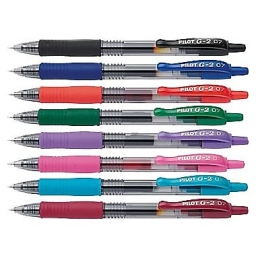# Individual 21713

They bought 22 sets of crayons for the school group. Larger sets cost CZK 65, smaller ones only CZK 50. They paid CZK 1,280 for all sets. How many individual types of crayon sets did they buy?

a =  12
b =  10

### Step-by-step explanation:

a+b=22
65a+50b=1280

a+b=22
65·a+50·b=1280

a+b = 22
65a+50b = 1280

Pivot: Row 1 ↔ Row 2
65a+50b = 1280
a+b = 22

Row 2 - 1/65 · Row 1 → Row 2
65a+50b = 1280
0.23b = 2.31

b = 2.30769231/0.23076923 = 10
a = 1280-50b/65 = 1280-50 · 10/65 = 12

a = 12
b = 10

Our linear equations calculator calculates it.Did you find an error or inaccuracy? Feel free to write us. Thank you!

Tips for related online calculators
Do you have a system of equations and looking for calculator system of linear equations?